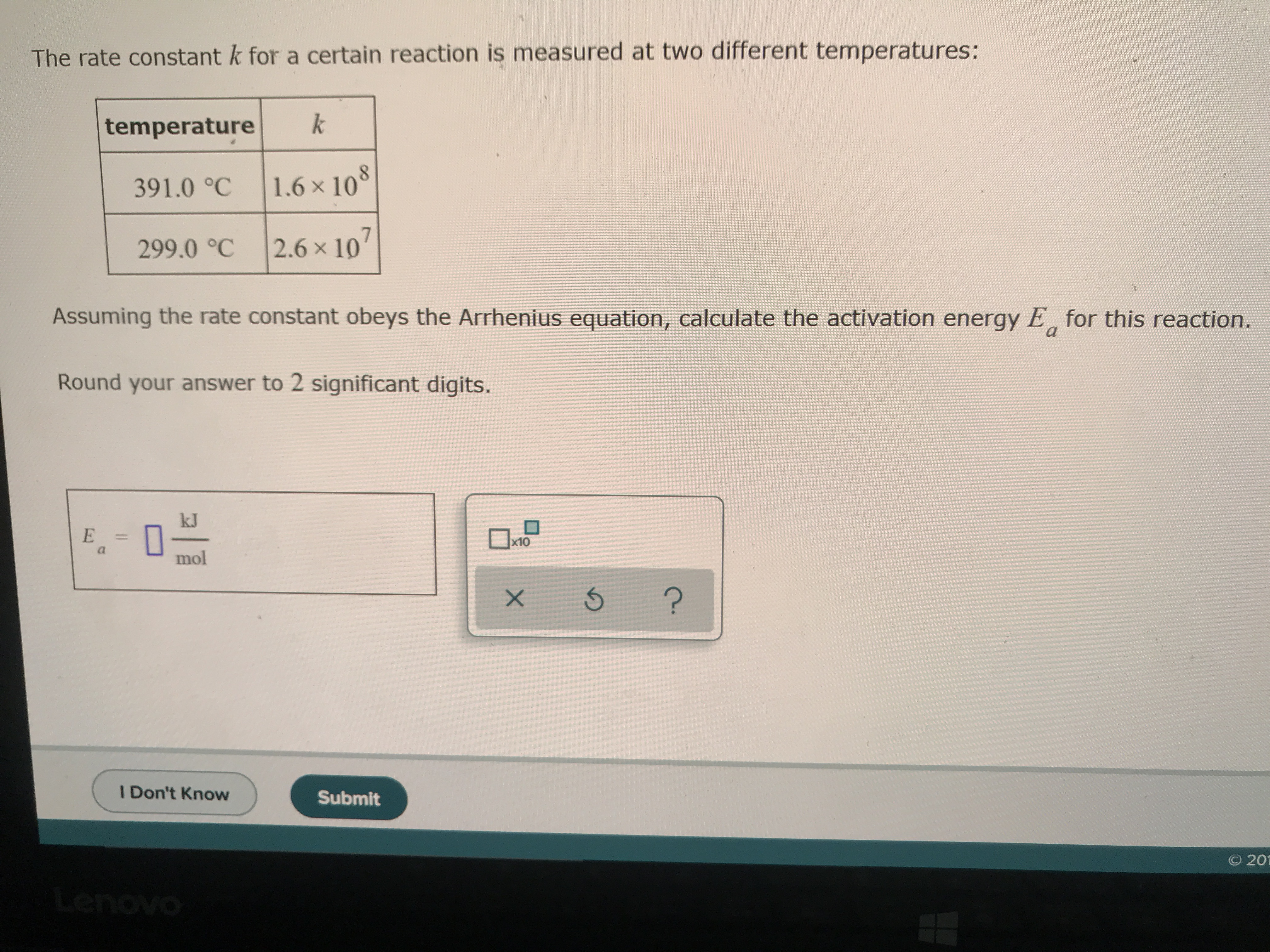# The rate constant, k, for a reaction is measured at two different temperatures (391.0 degrees Celcius: k =1.6x10^8, and 299.0 degrees Celcius: k = 2.6x10^7). Assuming the rate constant obeys the Arrhenius equation, calculate the activation energy, Ea, for the reaction. Round your answer to 2 significant digits.